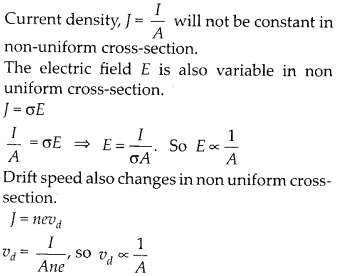Enlightened

# Question 18: NCERT Solutions for 12th Class Physics: Chapter 3-Current Electricity

• 0

Question 18: NCERT Solutions for 12th Class Physics: Chapter 3-Current Electricity

1. A steady current flows in a metallic conductor of non-uniform cross-section. Which of these quantities is constant along the conductor: current, current density, electric field, drift speed?
2. Is Ohm’s law universally applicable for all conducting elements? If not, give examples of elements which do not obey Ohm’s law.
3. A low voltage supply from which one needs high currents must have very low internal resistance. Why?
4. A high tension (HT) supply of, say, 6 kV must have a large internal resistance. Why?
Share

1. Solution:

1. The current will be constant because it is given to be steady.1. Ohm’s law is not a fundamental law in nature. It is not universally followed. Semiconductor diodes, transistors, their mistors, vacuum tubes etc. do not follow Ohm’s law.
2. If emf of supply battery is E and internal resistance r, then current through an external resistance R is given by
3.  so, internal resistance r should be least to supply high currents.
4. In high tension supply, the internal resistance is made large. Because, if accidently the short circuiting take place, the excessive current produced should not cross the safety limits.

Check the complete chapter with solutions.

NCERT Solutions for 12th Class Physics: Chapter 3-Current Electricity

• 0